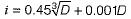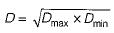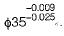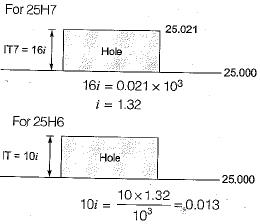Courses

# Test: Fits & Tolerance - 2

## 10 Questions MCQ Test Topicwise Question Bank for Mechanical Engineering | Test: Fits & Tolerance - 2

Description
This mock test of Test: Fits & Tolerance - 2 for Mechanical Engineering helps you for every Mechanical Engineering entrance exam. This contains 10 Multiple Choice Questions for Mechanical Engineering Test: Fits & Tolerance - 2 (mcq) to study with solutions a complete question bank. The solved questions answers in this Test: Fits & Tolerance - 2 quiz give you a good mix of easy questions and tough questions. Mechanical Engineering students definitely take this Test: Fits & Tolerance - 2 exercise for a better result in the exam. You can find other Test: Fits & Tolerance - 2 extra questions, long questions & short questions for Mechanical Engineering on EduRev as well by searching above.
QUESTION: 1

### In the tolerance specification, 25 D6 the letter D represents

Solution:

6 represents grade of tolerance and since D is used for hole it represents lower deviation (d used for shaft).

QUESTION: 2

Solution:
QUESTION: 3

### For sizes up to and including 500 mm, the tolerance unit i is determined from the equationWhich one of the following does D stands for

Solution:i.e. It is geometric mean of the 2 diameter step.

QUESTION: 4

Which one of the following is the value for the tolerance grade IT-8?

Solution:
QUESTION: 5

Tolerances are specified

Solution:
QUESTION: 6

According to Indian standard specifications, the total number of designated grades of fundamental tolerances are

Solution:
QUESTION: 7

Expressing a dimension as 25.3±0.05 mm is the case of

Solution:
QUESTION: 8

For the following hole and shaft sizes of mated parts according to basic hole system
Hole: 37.50 mm
37.52 mm
Shaft: 37.47 mm
37.45 mm
What is the value of allowance?

Solution:

Allowances = 37.50-37.47
= 0.03 mm

QUESTION: 9

A shaft has a dimension,The respective values of fundamental deviation and tolerance are

Solution:
QUESTION: 10

A small bore is designated as 25H7. The lower (minimum) and upper (maximum) limits of the bore are 25.000 mm and 25.021 mm, respectively. When the bore is designated as 25H8, then the upper (maximum) limit is 25.033 mm. When the bore is designated as 25H6, then the upper (maximum) limit of the bore (in mm) is

Solution:i.e. upper limit of bore = 25.013